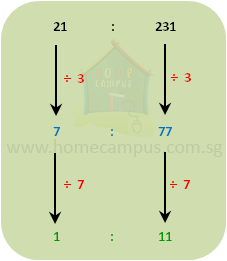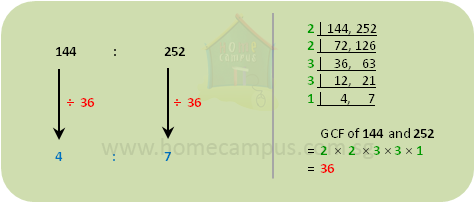## Simplifying Ratios

Practice Unlimited Questions

#### Why do we Simplify Ratios?

We simplify ratios to make the relation or comparison of quantities easier to understand.

The video tutorial above explains the concept in detail.

#### 1.  There are 21 horses and 231 chickens on a farm. Write the ratio of the number of horses to the number of chickens in the simplest form.

The ratio of the number of horses to the number of chickens is 21 : 231.

This tells us that for the 21 horses on the farm, there are 231 chickens.

However, to better understand the relation between the number of horses and the number of chickens, we should simplify this ratio as below.This simplified ratio 1 : 11 tells us that for every horse on the farm, there are 11 chickens.

So,    21 : 231  =  1 : 11    in its simplest form    (as we cannot divide the terms any further)

Method 1:
To simplify a given ratio, divide all the terms of the ratio by the same number until you cannot divide them any further.

#### 2.  Simplify 144 : 252 to its simplest form.

Method 2:
To simplify a ratio, you may divide the terms by their greatest common factor (GCF) as shown below.So,    144 : 252  =  4 : 7    in its simplest form.